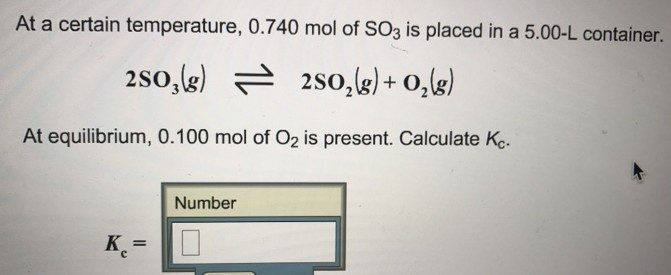# Problem: At a certain temperature, 0.740 mol of SO3 is placed in a 5.00-L container. 2SO3(g) ⇌ 2SO2(g) + O2(g) At equilibrium, 0.100 mol of O2 is present. Calculate Kc.

###### FREE Expert Solution
94% (453 ratings)###### Problem Details

At a certain temperature, 0.740 mol of SO3 is placed in a 5.00-L container.

2SO3(g) ⇌ 2SO2(g) + O2(g)

At equilibrium, 0.100 mol of O2 is present. Calculate Kc.